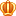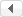##帐号 自动登录 找回密码 密码 立即注册
 搜索

# [平方十位数] 问题发表于 2019-1-28 22:02:09 | 显示全部楼层 |阅读模式
1鱼币
 我的疑问 程序的输出结果是一个 "哈哈", 证明  quareNumber() 函数返回了true, 但是在返回前还有一句cout语句却没有执行! 题目如下 由0~9这10个数字不重复、不遗漏，可以组成很多10位数字。 这其中也有很多恰好是平方数（是某个数的平方）。 比如：1026753849，就是其中最小的一个平方数。 请你找出其中最大的一个平方数是多少？ 注意：你需要提交的是一个10位数字，不要填写任何多余内容。 代码如下 #include #include #include using namespace std; // 检查是否存在重复的元素 bool repeat(int* array,int end) {         int num = {0};         for(int i = 0; i < end; i++)         {                 num[array[i]] += 1;         }         for(int i = 0; i < 10; i++)         {                 if(num[i] > 1) return true;         }         return false; } // 检查是不是平方数 bool quareNumber(int* array) {         long long sum = 0;         for(int i = 9; i >= 0; i--)         {                 sum += array[i];                 sum *= 10;         }         // 首先开方, 如果开方后的结果是个小数, 则这个数不是平方数         double a = sqrt(sum);         if(a == (int)a)         {                 cout<< a<< ":"<< sum;                 return true;         }         else false; } // array用来储存0-9的全排列 void dfs(int* array, int pos) {         if(pos == 10)         {                 if(quareNumber(array))                 {                         cout<< "哈哈";                         exit(0);                 }         }         for(int i = 9; i >= 0; i--)         {                 // 第一个数不能为0                 if(pos == 0 && i == 0) continue;                 // array中不能有重复的数字                 if(repeat(array, pos)) continue;                 array[pos] = i;                 dfs(array, pos+1);         } } int main(int argc, char* argv[]) {         int i;         dfs(i, 0);         return 0; } 复制代码 执行结果 哈哈 -------------------------------- Process exited with return value 0 Press any key to continue . . .发表于 2019-1-28 22:23:12 | 显示全部楼层
 请查看代码注释： // 检查是不是平方数 bool quareNumber(int* array) {         long long sum = 0;         for(int i = 9; i >= 0; i--)         {                 sum += array[i];                 sum *= 10;         }         // 首先开方, 如果开方后的结果是个小数, 则这个数不是平方数         double a = sqrt(sum);         if(a == (int)a)         {                 cout<< a<< ":"<< sum;                 return true;         }         //else false;  //修改为如下：         else         {                 return false;         } }复制代码

 本版积分规则 回帖后跳转到最后一页

## 小甲鱼强烈推荐/1GMT+8, 2019-8-25 04:19

Powered by Discuz! X3.4

© 2001-2017 Comsenz Inc.